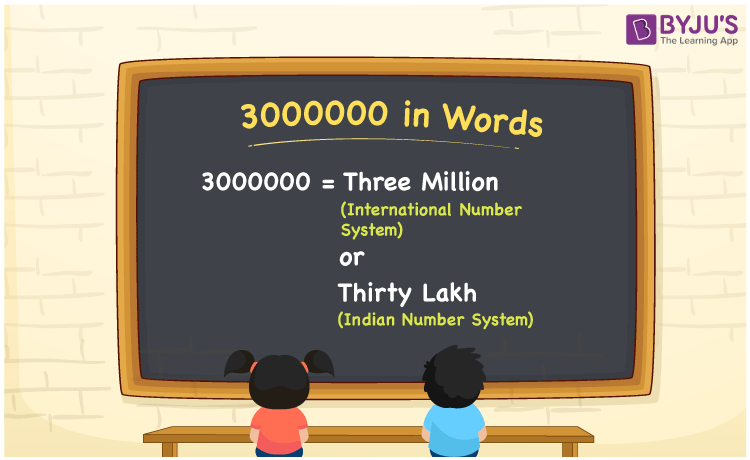# 3000000 in Words

We can express 3000000 in words as Three Million (in International Number System) or Thirty Lakh (in Indian Number System). If you purchased a house worth Rs. 3000000, then you can say, “I have purchased a house worth Rupees Thirty Lakh. 3000000 is a cardinal number. The number name is generally represented using ones, tens, hundreds, thousands etc. Let us learn to write 3000000 in words with the help of a place value chart.

 3000000 in words International Number System Indian Number System Three Million Thirty Lakh Three Million or Thirty Lakh in numerical form 3000000

## 3000000 in English Words

We know that generally English alphabet is used to convert numbers in words. Hence, 3000000 in words or the spelling of 3000000 in English is Three Million or Thirty Lakh.## How to Write 3000000 in Words?

The place value chart helps to write 3000000 in words. Hence, 3000000 is represented in the place value chart as below:

 Ten Lakhs Lakhs Ten-Thousands Thousands Hundreds Tens Ones 3 0 0 0 0 0 0

Hence, we can write this in expanded form as:

3 x Ten Lakh + 0 x Lakh + 0 x Ten Thousand + 0 x Thousand + 0 x Hundred + 0 x Ten + 0 x One

= 3 x 1000000 + 0 + 0 + 0 + 0 + 0 + 0

= 3000000

= Three Million or Thirty Lakh

Therefore, 3000000 in words is written as Three Million or Thirty Lakh.

Interesting way of writing 3000000 in words

3 = Three

30 = Thirty

300 = Three Hundred

3000 = Three Thousand

30000 = Thirty Thousand

300000 = Three Lakh

3000000 = Three Million or Thirty Lakh

Hence, the word form of the number 3000000 is Three Million or Thirty Lakh.

3000000 is a natural number that is succeeded by 2999999 and preceded by 3000001

• 3000000 in words – Three Million or Thirty Lakh
• Is 3000000 an odd number? – No
• Is 3000000 an even number? – Yes
• Is 3000000 a perfect square number? – No
• Is 3000000 a perfect cube number? – No
• Is 3000000 a prime number? – No
• Is 3000000 a composite number? – Yes

## Frequently Asked Questions on 3000000 in Words

Q1

### How to write 3000000 in words?

3000000 in words is written as Three Million or Thirty Lakh.
Q2

### Calculate 14300 plus 2985700 and express in words.

14300 + 2985700 = 3000000 Thus, 3000000 in words is Three Million or Thirty Lakh.
Q3

### Is 3000000 a prime number?

No, 3000000 is not a prime number.